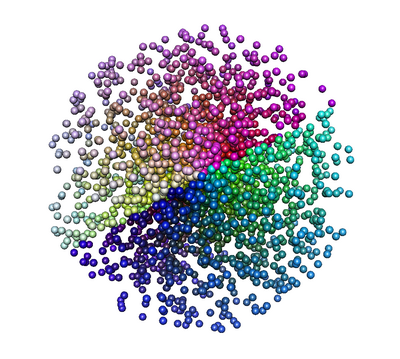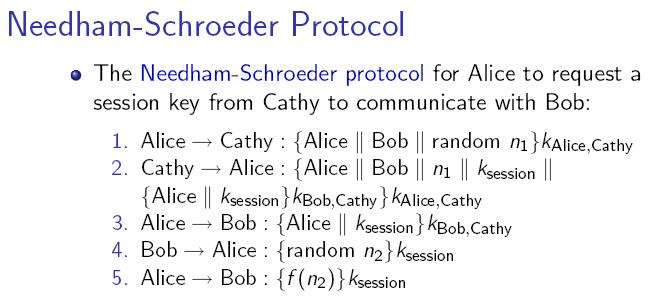# Random Number Generators and Information Security

A random number generator (often abbreviated as RNG) is a device that generates a sequence of numbers without any discernible pattern. A RNG must pass certain statistical tests to be considered valid. There are many applications of randomness, such as gambling, game simulation and, of particular interest to this wikipage, cryptography. The wide use for random numbers has led to many different methods for their generation, but they all fall under one of two categories: physical or computational. Both have advantages and disadvantages, depending on whether efficiency or security is the top priority.## Relevance to Information Security

Random numbers are used in many aspects of computer science. This wiki page discusses the particular relevance of random numbers with information security -- its applications in cryptography.
For example, the Needham-Schroeder Protocol includes random numbers in the message to protect data integrity. A RNG that gives predictable output would make the system vulnerable to replay attacks. Since a system is only as secure as its weakest component, random number generators are a crucial part of information security.## Vulnerability to attack

The RNG process is usually a single isolated component easy to locate, making it an attractive target for attackers. If an attacker can predict the sequence of supposedly random numbers, data integrity and confidentiality are compromised. An attack on RNG is difficult to detect by any upstream test of the numbers. Furthermore, such attacks require only a single access to the system. No data need be sent back in contrast to, for example, a computer virus that steals keys and then e-mails them to some drop point.Phishing

For example, the RANDU random number algorithm used for decades on mainframe computers was seriously flawed, and as a result a lot of research work of that period is less reliable than it might have been.

Microsoft uses the CryptGenRandom function to generate random values for its Windows operating system. This function is included in Microsoft's Cryptographic Application Programming Interface, and is used whenever random numbers are needed. It is said to be crytographically secure, but the specifics of the algorithm have not been officially published and verified. Researchers have used reverse engineering to test it and have discovered security concerns. An attacker needs only to steal the state bits once, and then they can persistently violate the security of a CryptGenRandom instance and even determine past random numbers generated. This is a serious problem, since the process can be run backwards once the state bits are known, and information already sent are compromised. For example, if the user has made online purchases from websites such as eBay, the random key used to encrypt credit card information can be recovered by an attacker. Moreoever, the CryptGenRandom function runs in user mode, so anyone with access to a regular user account on a system can easily obtain access to important information such as the state bits.

## Two kinds of randomness

### True randomness - Physical methods

The earliest methods for generating random numbers - dice, coin flipping, roulette wheels are still used today, mainly in games and gambling as they tend to be too slow for applications in statistics and cryptography. Some physical phenomena, such as thermal noise in zener diodes, appear to be truly random and can be used as the basis for hardware random number generators.

The generation of true random numbers through measuring physical phenomena is based on the theory of entropy. For example, HotBits is an online generator of true random numbers, using timing successive pairs of radioactive decays detected by a Geiger-Müller tube interfaced to a computer. This process is governed by the inherent uncertainty in the quantum mechanical laws of nature. RANDOM.ORG, on the other hand, looks at variations in the amplitude of atmospheric noise. Even lava lamps have been used to generate random numbers -- images of the lamps are converted into an unique video stream.

### Pseudorandomness - Computational methods

Computational methods use mathematical formulae or simply precalculated tables to produce sequences of numbers that appear random. Since the computer follows a deterministic algorithm to generate these numbers, they are inherently predictable.

For example, Blum Blum Shub (BBS) is a pseudorandom number generator proposed in 1986 by Lenore Blum, Manuel Blum and Michael Shub. It generates sequences of random numbers with the following formula: xn+1 = (xn)2 mod M, where M = pq, p and q are both prime numbers. The developers of this method have proved that it is extremely secure. To crack this algorithm would essentially require factorization of large primes, which is assumed to be mathematically infeasible. With a large M, the output of BBS displays no nonrandom patterns that can be discovered with a reasonable amount of calculation.

### Verdict

Physical Methods are:

• Slower than computational methods, since sequences of random numbers are generated in real-time through measuring physical phenomena.
• Nondeterministic.
• Truly unpredictable; this characteristic "can be traced to the laws of quantum mechanics", which are unpredictable as we know them.

Computational Methods:

• Efficient: long sequences of numbers can be produced very quickly.
• Deterministic: given the seed state, any sequence of numbers can be reproduced anytime. This can be an advantage if past sequences are needed at a later date for validation or analysis purposes. However, it may make the system vulnerable to replay attacks (see Vulnerability to Attacks sectiona bove).
• Periodicity: all sequences eventually repeat themselves. Despite sounding dubious, this characteristic does not present a practical problem, as the periods of most PRNG's used today are very large and thus sequences do not actually repeat in realistic situations.

The basic difference between PRNGs and TRNGs can be explained with the analogy of rolling a die. PRNGs produce sequences of numbers using mathematical formulae or precalculated lists (ie. someone performed many trials and recorded the results), and output numbers from the sequences (ie. the next number on the pre-recorded list). The numbers appear random, but they are really predetermined. If an attacker obtains the algorithm (the list of numbers) and the seed state (the starting number), he or she can predict every "random" number in the sequence.
TRNGs measure physical phenomena (ie. actually rolling the die and reading out the number). They are as unpredictable as the physical phenomenon used. Even if the attacker knows how the numbers are generated and obtains a record of past numbers, he or she cannot predict the next number with any certainty.
Both methods have their advantages and disadvantages. When efficiency is a priority, such as in simulation and modeling applications, computational methods are more appropriate. When unpredictability is crucial, such as in the case of data encryption, physical generators are generally preferred.

## Conclusion

To fully understand information security, we must understand how each component of the system works. Random number generators are an important part of any information security system because of their relevance in cryptography. To protect data integrity and confidentiality better, a RNG that produces fast and truly unpredictable random numbers is crucial. The RNGs in use today all have advantages and disadvantages. They are far from perfect and are still being improved today.

## References

1. Bishop, M. 2004. Introduction to Computer Security. Prentice Hall PTR.
2. Wikipedia: Random Number Generation. 2007. Retrieved November 27, 2007.
3. Wikipedia: Random number generator attack. 2007. Retrieved November 27, 2007.
4. Haahr, M. 2007. Random.org. Retrieved November 27, 2007. Random.org
5. California Soil Resource Lab. 2007. Retrieved November 27, 2007.
6. HotBits. 2007. Retrieved November 27, 2007.
7. Wikipedia: Blum Blum Shub. 2007. Retrieved November 27, 2007.
8. Lavarnd. 2007. Retrieved November 27, 2007.

--Caoff 11:58, 09 December 2007 (EST)